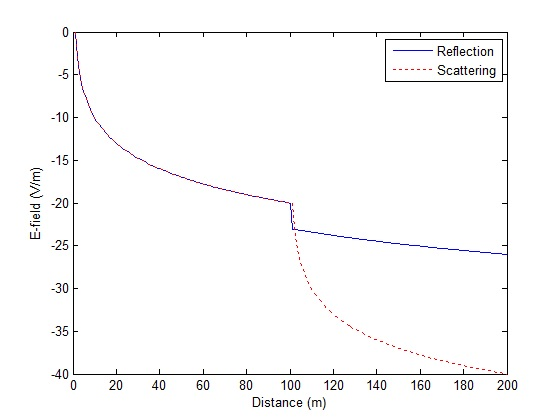Reflection vs Scattering in Ray-Tracing

In ray-tracing simulations one is faced with a complexity at points of intersection between rays and objects. How to handle the reflection, should it be specular or scattered. In this post we consider the two extreme cases; pure reflection vs scattering.

For this we consider that in the case of specular reflection the power of the ray is reduced by 3dB (that is R=0.5) and the ray continues as it would if there was no interaction with the object (off course direction would be changed with angle of reflection being equal to angle of incidence).

In the case of scattered ray we assume that the point of interaction between the ray and the object is a point source from where the rays are regenerated. Therefore there is a rapid drop in the E-field strength as the ray propagates away from the point of interaction.

```clear all
close all

Eo=1;
r1=1:100;
r2=101:200;
r=[r1 r2];
R=0.5;

Er1=Eo./r1;
Er2=R*Eo./r2;
Er=[Er1 Er2];

Es1=Eo./r1;
Es2=Es1(end)./(r2-r1(end));
Es=[Es1 Es2];

plot(r,10*log10(Er),'b')
hold on
plot(r,10*log10(Es),'r:')
hold off

legend('Reflection','Scattering')
xlabel('Distance (m)')
ylabel('E-field (V/m)')
```The simulation code above considers that a ray travels unobstructed for 100 m and at this point comes in contact with an object and is reflected (reflection coefficient of 0.5 is assumed) or scattered. The ray then again travels for another 100 m without coming in contact with an object.

It is observed that there is a rapid decrease in E-field strength of the scattered ray beyond 100 m. This is because the E-field strength at the point of interaction is much lower than that at the source and when this is considered to be a point source, re-generating the rays, the resulting E-field decays quite rapidly.

We assume that the reflection is specular in most of our simulations as this is closer to reality than assuming a fully scattered ray.Author: John (YA)

John has over 15 years of Research and Development experience in the field of Wireless Communications. He has worked for a number of companies around the world including Qualcomm Inc. USA. He has an MS in Electrical Engineering from Virginia Tech USA and has published his work in international journals and conferences.

0.00 avg. rating (0% score) - 0 votes

3 thoughts on “Reflection vs Scattering in Ray-Tracing”

1.John says:

It goes in all of the directions, since the point of intersection of the ray and the object is considered to be a new source.

2.Sergey says:

Nice and elegant model. However, where does the scattered light go?

Can it be accounted for via creating a new bundle of rays originating from the point of contact? Then it seems that roughness should be proportional to the random phase wobble of the new rays relative to the old one.

Best regards